Amazon SageMaker is the machine learning platform of AWS which helps solve the different requirements of data scientists and machine learning practitioners. It has several features and capabilities that assist in the different stages of the machine learning process. Here is a simplified list of the capabilities of SageMaker mapped to some of the stages of the ML lifecycle.

 SageMaker Processing Data Preparation and Processing SageMaker Training Model Training SageMaker Automatic Hyperparameter Tuning Model Training SageMaker Debugger Model Training SageMaker Hosting Services Deployment and Monitoring SageMaker Model Monitor Deployment and Monitoring

There’s definitely more into this list which we will not include and discuss here!

In this 2-part tutorial, we will focus on SageMaker Processing and how we can use it to solve our data processing needs. Our overall goal is to demonstrate how to use SageMaker Processing to help us perform Min-Max scaling on a dataset in its own dedicated and easily scalable environment. If you are looking for Part 2, you can find it here.We will generate a sample dataset and perform Min-Max scaling on this dataset. This sample dataset will just have 10 records to make it easy for us to demonstrate how things work from start to finish. Once we are ready to deal with significantly larger datasets and files, the same solution using SageMaker Processing in this tutorial can be already used with minimal modifications.

## Concepts

What’s Min-Max scaling? Min-Max scaling normalizes and transforms the input variable values into a new set of values with the following properties:

• New maximum number = 1
• New minimum number = 0
• The maximum value from the original list gets the value of 1
• The minimum value from the original list gets the value of 0

Min-Max scaling makes use of the following formula to compute for the new value: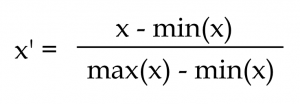Here is a quick example of how this formula is used:

Original

 1 2 5 4 3

Scaled

 0 0.25 1 0.75 0.5

Why is this important? The scale across the different features of the dataset affects the performance of certain models trained using this dataset. This includes SVM and linear regression for example. On the other hand, decision trees are not affected by the differences in the scale of the input variable values.

Note that we are not limited to just using Min-Max scaling with SageMaker Processing. Since we are going to write our own custom code, we can easily just make use of the available libraries already installed inside the container environment where the script is running. Later, we will be using the SKLearnProcessor class from the SageMaker Python SDK. This will help us run our custom Python script inside a pre-built container image with scikit-learn already installed. This means that we can also use the following from the scikit-learn package:

• RobustScaler
• OneHotEncoder
• StandardScaler

In case you are wondering what else we can do with SageMaker Processing, you should know that we can technically do anything we want with the data using scikit-learn and the other Python libraries inside the running container. Given that we are given a blank canvas with a custom script, we can also do other things such as model evaluation and data format transformation with this approach.

In this tutorial, we will use the following libraries and tools:

If this is your first time using one or more of these, do not worry as we will get a better understanding of how these are used later in this tutorial.

### Prerequisites

Now that we have a better understanding of what we are trying to accomplish, we can now proceed with the step-by-step tutorial on how to get this working inside SageMaker. We will divide this tutorial into 4 sections:

 Synthetic Data Generation

 Using MinMaxScaler without the managed infrastructure support of SageMaker Processing

 Using SageMaker Processing with local mode (found in PART II)

 Using SageMaker Processing with dedicated ML instances (found in PART II)

Before we start, make sure that you have:

(1) An AWS Account

(2) A running SageMaker notebook instance. You may use SageMaker Studio but note that we will not be able to use local mode in SageMaker Studio. Later, we will explain what this is and how this helps us test our scripts and experiments first before deciding to use dedicated ML instances. If this is your first time using SageMaker, feel free to follow the steps here: https://docs.aws.amazon.com/sagemaker/latest/dg/nbi.html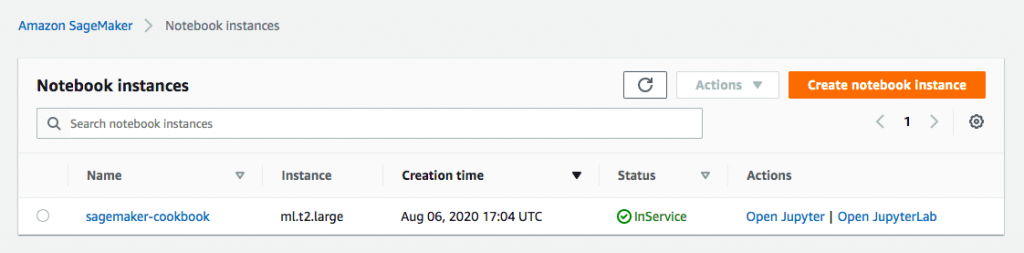Once you have a running notebook instance, click Open Jupyter. Inside the Jupyter app, create a new notebook using the conda_python3 kernel.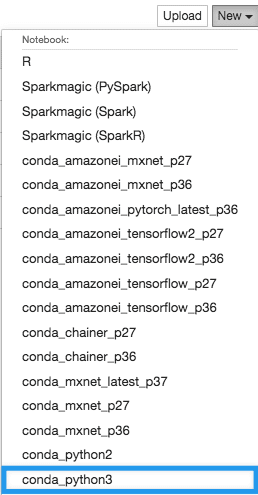We will use this Jupyter notebook to run the succeeding code blocks in this tutorial.

### Section I. Synthetic Data Generation

In this section, we will generate a sample dataset containing 10 records. The expected output here would be a DataFrame with 3 columns: x1, x2, and y. Run the following steps and blocks of code inside our Jupyter Notebook.

1. Define the 3 functions which will help us generate a list of random numbers for our dataset.

```import random def generate_x1_values(count=10):   return random.sample(range(10, 100), count) def generate_x2_values(count=10):   return random.sample(range(-1000, 1000), count) def generate_y_values(x_values):   return [x * 2 for x in x_values]```

1. Generate the list of values for the x1 column

```x1 = generate_x1_values() x1```

This should give us a structure containing random numbers similar to [66, 35, 34, 78, 14, 26, 72, 61, 62, 33]. Here, we can see that the numbers in this list are within the [10, 100] range.

1. Generate the list of values for the x2 column

`x2 = generate_x2_values() `

`x2`

This should give us a structure containing random numbers similar to [432, 113, 55, 170, 383, -548, -210, 571, 250, -858]. Here, we can see that the numbers in this list are within the [-1000, 1000] range.

1. Generate the list of values for the y column

`y = generate_y_values(x1) `

`y`

This should give us a structure similar to [132, 70, 68, 156, 28, 52, 144, 122, 124, 66]

1. Prepare the DataFrame using the lists we have prepared in the previous steps

```import pandas as pd df = pd.DataFrame({   "x1": x1,   "x2": x2,   "y": y }) df```

This should give us a DataFrame similar to what is shown in the following image: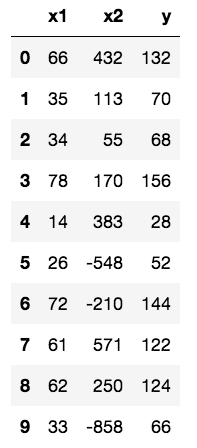You might be wondering what’s x1 and you might also be wondering what’s y. In a real dataset, y might be the price of an item, x1 might be the item’s length, and x2 might be the item’s weight. In a complete machine learning experiment, we might be trying to predict the value of y using the values of x1 and x2.

Now that we have our synthetic dataset, we will proceed with the sections that normalize the data using 3 different approaches.

## Section II. Using MinMaxScaler without the managed infrastructure support of SageMaker Processing

In this section, we will see how MinMaxScaler is used without SageMaker Processing. Run the following steps and blocks of code inside the same Jupyter Notebook as with the previous section.

1. Initialize the MinMaxScaler object and use the fit_transform() method to

```from sklearn.preprocessing import MinMaxScaler scaled_df = df.copy() scaler = MinMaxScaler() scaled_df[["x1", "x2", "y"]] = scaler.fit_transform(df[["x1", "x2", "y"]]) scaled_df```

This should give us a DataFrame of values similar to what is shown in the following image: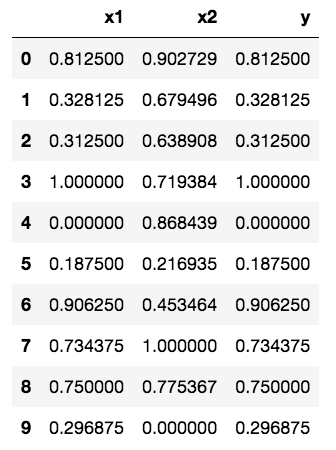You can see in the previous image that the smallest value is 0 and the largest value is 1. Now that we are done testing the MinMaxScaler from scikit-learn, we will make use of similar blocks of code in the next section (which can be found in the 2nd part of this tutorial).

### What’s next?

Now that we’re done with Part I, we can now proceed with Part II which focuses on how we can use SageMaker to process our data. You can find Part II here.

If you want to dig deeper into what Amazon SageMaker can do, feel free to check the 762-page book I’ve written here: https://amzn.to/3CCMf0S. Working on the hands-on solutions in this book will make you an advanced ML practitioner using SageMaker in no time.In this book, you should find the different ways on how to configure and use SageMaker Processing:

• Installing and using libraries within the custom Python script
• Using custom container images with SageMaker Processing
• Passing arguments from the Jupyter notebook and reading the arguments within the custom script
• Creating automated workflows with Step Functions and SageMaker Pipelines which utilize SageMaker Processing for the data preparation and transformation step

You should find all the other features and capabilities of SageMaker such as SageMaker Clarify, SageMaker Model Monitor, and SageMaker Debugger here as well.

That’s all for now and stay tuned for more!

#### FREE AWS, Azure, GCP Practice Test Samplers